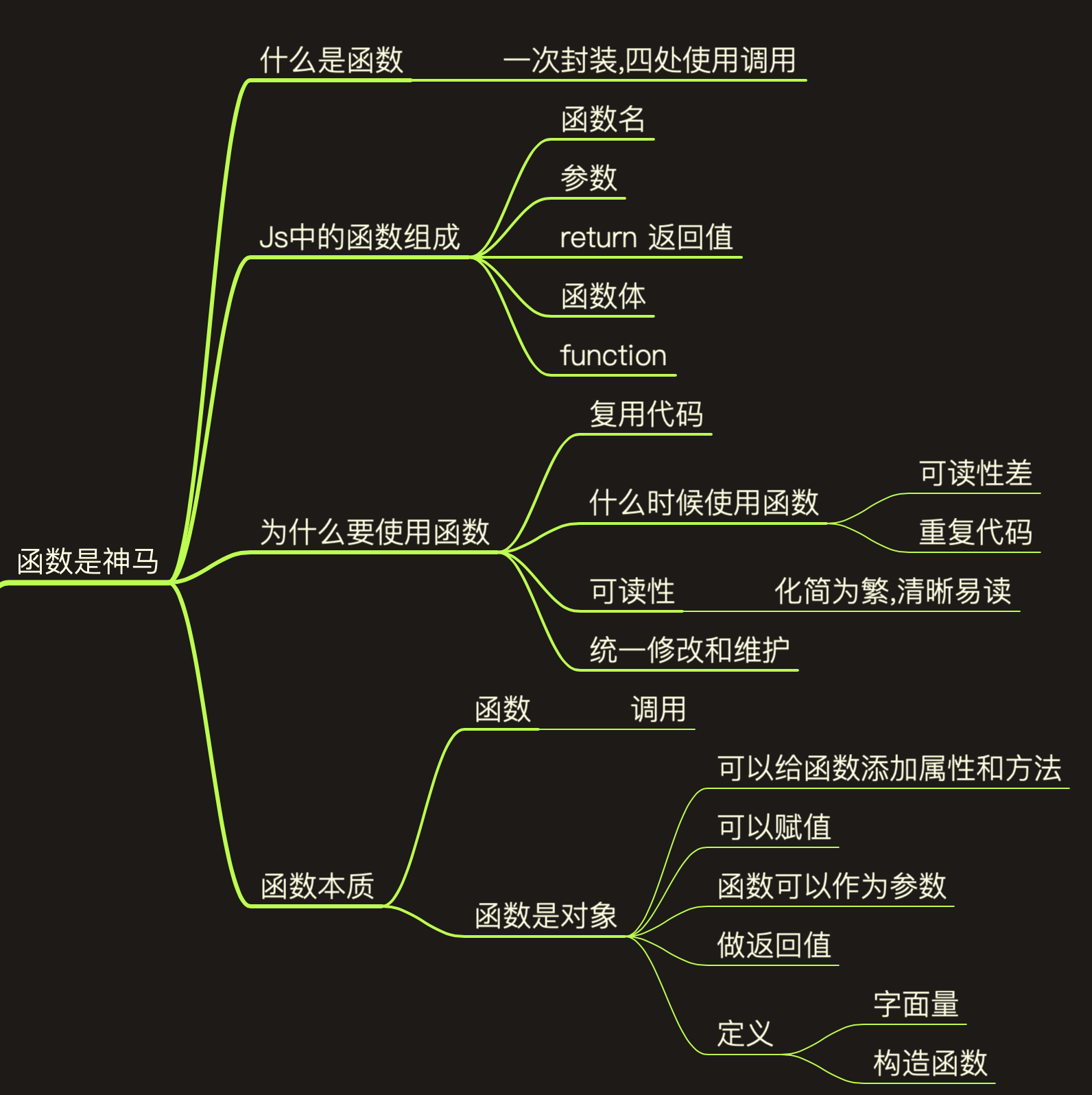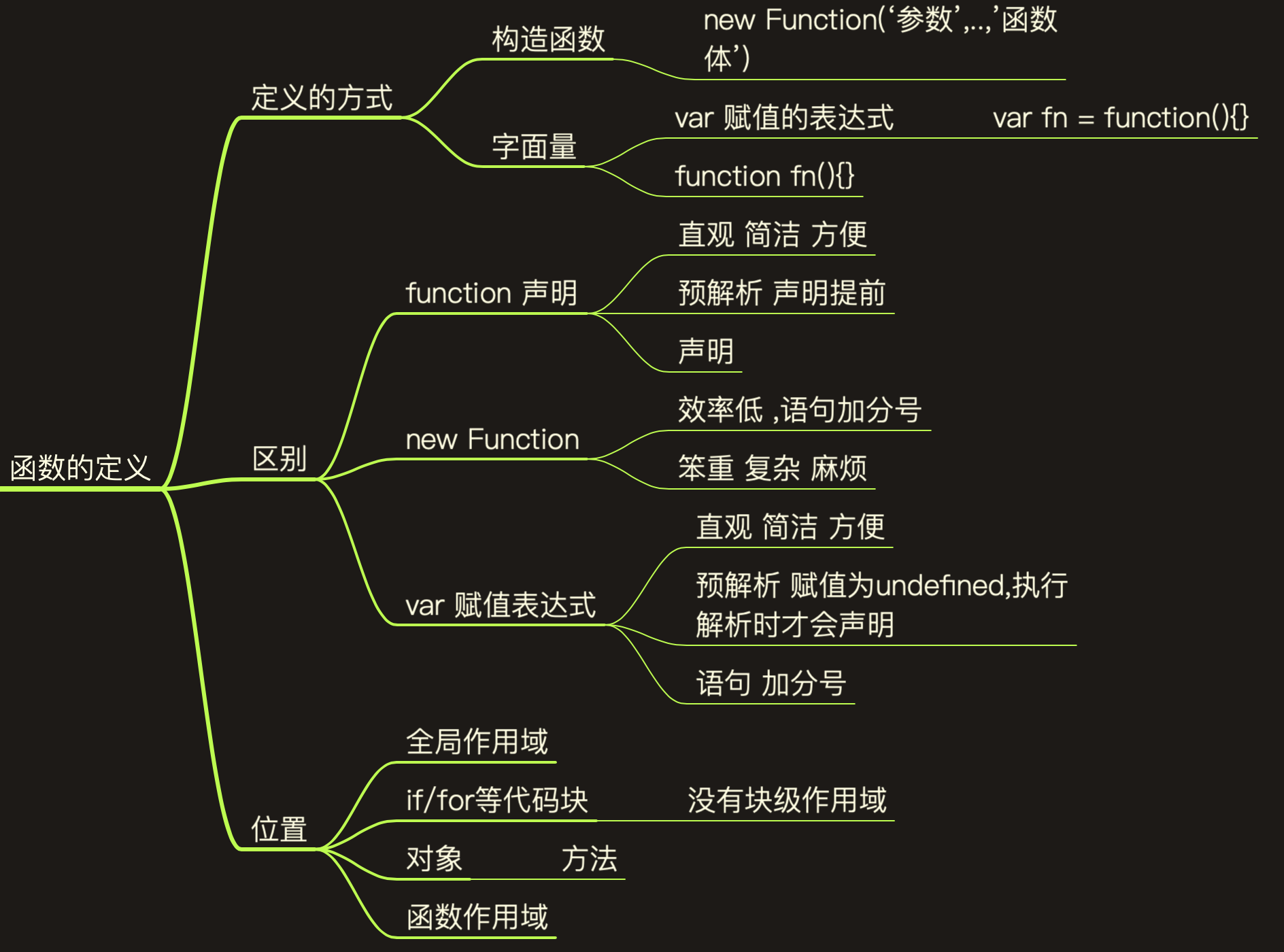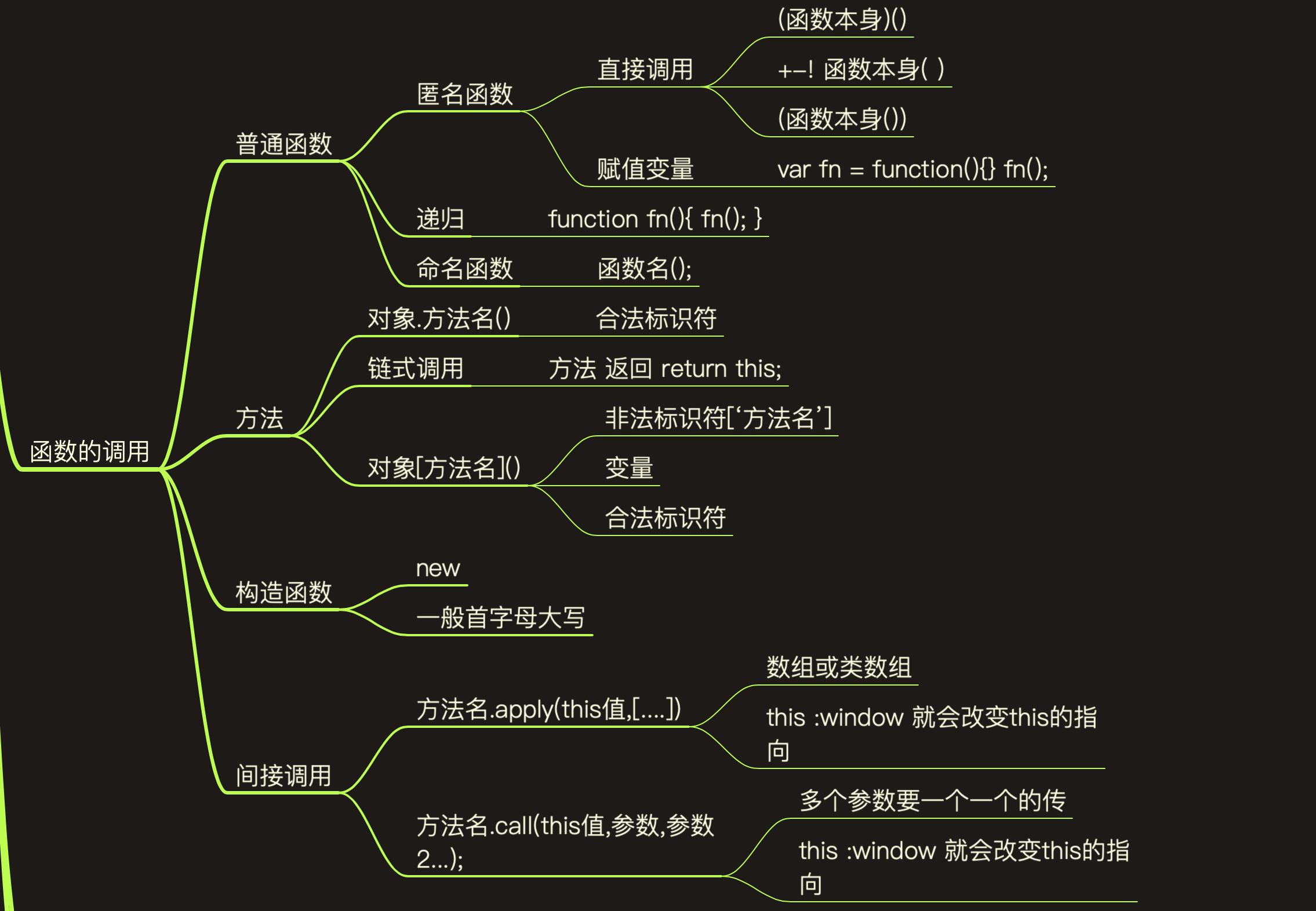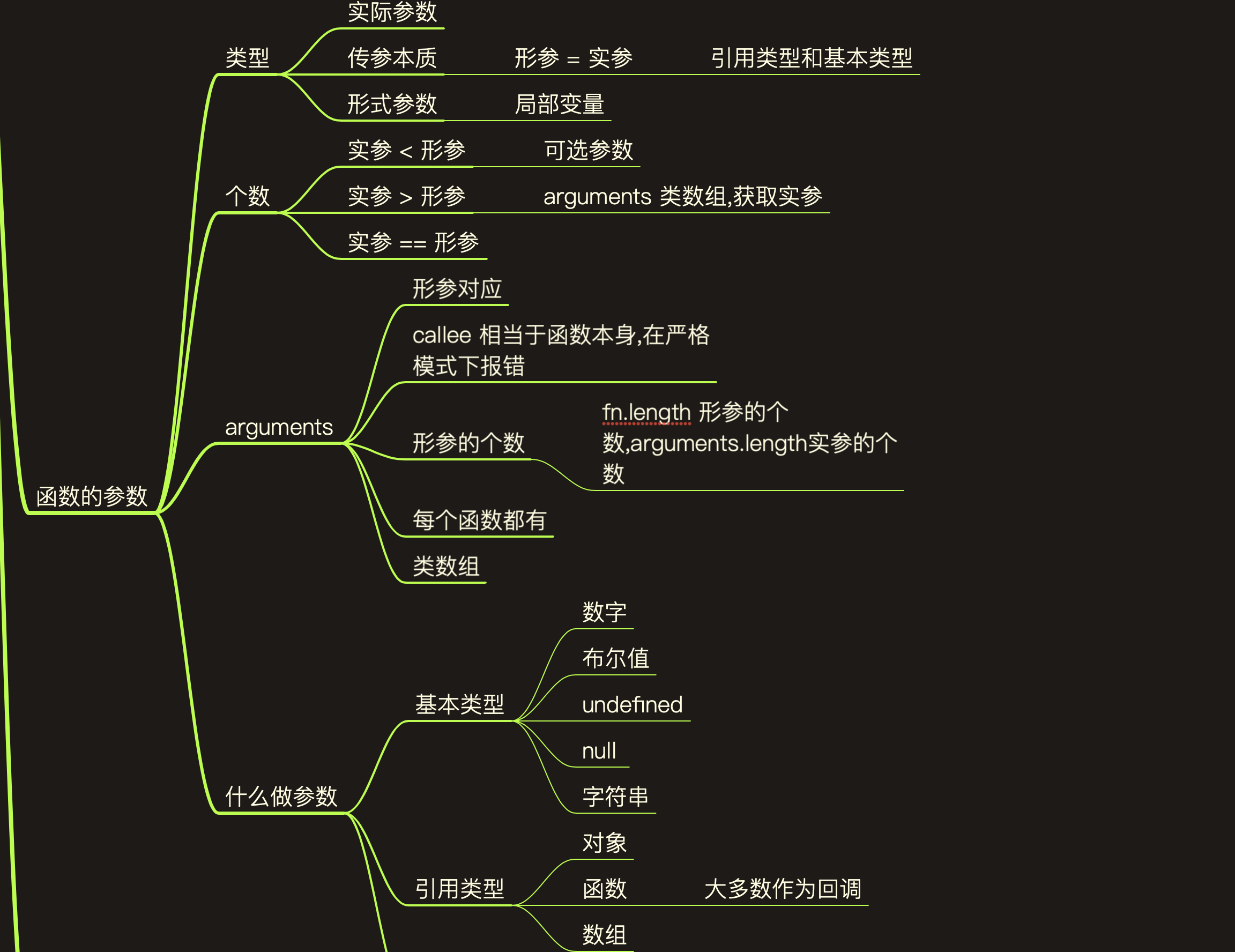# JavaScript 函数

| 阅读### 函数是神马``````       console.log(add(1, 2));
/**
* 函数可以复用代码
*/
function add(num1, num2) {
return num1 + num2;
}
``````

`````` //JavaScript 中函数就是对象
function add(num1, num2) {
return num1 + num2;
}
//给函数添加属性和方法
add.setSex = function (sex) {
this.sex = sex;
}
``````

``````      var text = function () {
return "atex";
}
text();
console.log(text());//atex
console.log(text);//function(){return "atex"}
//数组中可以 对象、函数
[{}, function () { }, { family: {}, setName: function (name) { } }]
//函数可以作为参数使用
//函数名加()表示函数被执行,不加参数表示将函数的本体传入
``````

``````       //函数作为返回值
function fn() {
return function () {
console.log(1);
}
}
var newFn = fn();
//调用函数返回值的函数
newFn();//1
fn()();//1
``````

``````//在预解析的时候就已经对声明函数加载了 并不能达到if/else按需 定义函数的作用
if (true) {

}
} else {
function sub() {

}
}
``````

``````//可以使用下面的方式,使if/else起到作用,因为 add sub 是变量,在预解析时就变为了undefined,但是并不推荐使用这种方式
if (true) {
var add = function () {

}
} else {
var sub = function () {

}
}
``````

`````` //匿名函数头部需要存在合法的值 才可以自执行
//匿名函数定义直接执行
var s = function () {
console.log('');
}();

//匿名函数的自执行
(function () {

})();
(function () {

}());
//匿名函数也可以这样用
!+function () {
console.log('1');
}();
//也可以这样用
console.log(function () {
return 1;
}());

``````

``````/递归调用
//计算阶乘
function fn1(num) {
if (num <= 1) return 1;
return num * fn1(num - 1);
}

console.log(fn1(5));

//方法的调用
var option = {
add: function (num1, num2) {
return num1 + num2;
},
sub: function (num1, num2) {
return num1 - num2;
}
}
var key = 'add';
option[key](2, 2);

//链式调用
var option = {
add: function (num1, num2) {
console.log(num1 + num2);
return this;
},
sub: function (num1, num2) {
return this;
}
}
``````

``````//构造函数的调用

function Person(){

}
//是不是通过new 来调用构造函数
var obj = new Person();

//间接调用

// }
// //每个函数下都有两个方法

var name = 'xm';
var person = {};
person.name = 'xh';

person.getName = function(){
return this.name;
}

console.log(person.getName());//xh
console.log(person.getName.call(window));//xh call 改变this的指向 this不指向了person 而是指向window

return num1 + num2;
}
var data = [1,2];
``````

`````` //动态设置属性
var person = {
setPerson: function (property, value) {
person[property] = value
}
};

person.setPerson('name', 'xm');
person.setPerson('age', 18);
person.setPerson('sex', 'male');
``````### 函数的参数

``````   function add(num1, num2) {
return num1 + num2;
}
add(1);//num1 = 1 ; num2 = undefined
//可选参数
function pow(base, pow) {
pow = pow || 2;
return Math.pow(base, pow);
}
console.log(3);
console.log(3,2);
``````

``````var arguments =  {
'0':1,
'1':2,
'length':2
}
``````

``````        function add(){
if(arguments.length === 0) return;
var nums = 0;
for (let index = 0; index < arguments.length; index++) {
const element = arguments[index];
nums += element;
}
return nums;
}
``````

``````       function fn(name){
arguments = '';
console.log(name);//'' name值也发生变化了
function fn2(){//每个函数都有一个独特的arguments
console.log(arguments);//fn2 的自己的arguments
}
}
``````

``````  //arguments.callee 函数的本体 可以用到在递归调用中
//场景如下:
//如果函数jiecheng 内部过于复杂,用到了递归,某天改掉了函数名,还要将每个递归调用的函数名都要改掉
//用arguments.callee 就是当前函数的本体,这样就不用在手动去改掉函数名了.
// "use strict"//严格模式下 不允许使用arguments.callee 如何修改呢?
function jiecheng(num){
if(num <= 1) return 1;
return num * arguments.callee(num - 1);
}

console.log(jiecheng(5));

严格模式下 可以这样使用
var jiecheng =  function fn(num){
if(num <= 1) return 1;
return num * fn(num - 1);
}
console.log(jiecheng(5));
``````